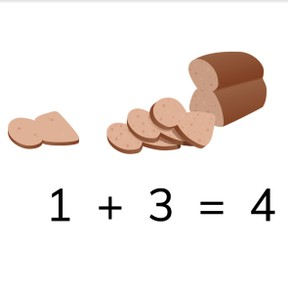# Recognizing addition problems to 5

I can recognize addition problems using pictures to 5.

No account needed.8,000 schools use Gynzy92,000 teachers use Gynzy1,600,000 students use Gynzy

-K.OA.A.1
-K.OA.A.2

## Relevance

Discuss with students that you can use images and objects to determine which addition problems belong to them. This demonstrates how addition problems work and say that you need this to learn how to do addition.

## Introduction

Show the foxes on the interactive whiteboard and ask the students to count the foxes. Repeat this with the beads and stripes on the interactive whiteboard.

## Development

Show the blocks of cheese on the interactive whiteboard. There are blocks with flags, and without flags. First count the blocks with the flags, and then those without flags. There is 1 block with a flag, and one without. These blocks without flags are added to the block you already had. The addition problem is 1 + 1. Next you show one block of cheese with a flag and two without flags. You count the one block with, and two without together. That is 1 + 2. Discuss all the variations of these shown on the interactive whiteboard and show the accompanying addition problem. Next, you show them the cars. Say that you first count 3 cars, and count that there are 2 cars in the 2nd box. Emphasize that the + sign goes between them because you want to add them together. Say that the first number is the first one you see, and the second number is the one that is added to the first.
Next show the students a wheelbarrow with pumpkins. Ask students if they can figure out what the addition problem is. Next you must drag coins to the piggy banks. The addition problem is shown, and students must decide how many coins must be dragged, and if they belong above or in the piggy bank. Explain, using the cars, that you find the sum, or total by counting the two groups together. Practice this using the lake with frogs to find the addition problems and their solutions. You can erase the grey boxes to show the answers.

Check that students understand recognizing addition problems using pictures to 5 by asking the following questions.
- How do you know which numbers create an addition problem?
- What should you do if you want to know what two groups together are?
- How do you know which number is first in an addition problem?
- How do you know what the second number is in an addition problem?

## Guided practice

Students first practice using images to recognize addition problems from the multiple choice answers given. Next they must not only recognize, but also solve the addition problems.

## Closing

Discuss with students that it is important to be able to recognize which images belong to an addition problem so that you can understand how addition problems work. Ask students to work in pairs and practice addition. The first student writes an addition problem, and the second must draw images to match the problem. They then must solve the problem together. They then switch.

## Teaching tips

Students who have difficulty using the images to represent addition problems can be supported by the use of MAB-blocks. Set one group of blocks on the left, the other on the right, and ask the student to count the blocks (per group) one by one.

### The online teaching platform for interactive whiteboards and displays in schools

• Save time building lessons

• Manage the classroom more efficiently

• Increase student engagement